## Methods of a Fully Automated CONSORT Diagram Macro %CONSORT

Started ‎03-15-2021 by
Modified ‎11-23-2021 by
Views 3,104
Paper 1161-2021
Author

Jeffrey Meyers, Mayo Clinic

## Abstract

The CONSORT diagram is commonly used in clinical trials to visually display the patient flow through the different phases of the trial, and to describe the reasons patients dropped out of the protocol schedule. They are very tedious to make and update as they typically require using different software, such as Microsoft Visio or Microsoft PowerPoint, to manually create, align and enter the values for each textbox. There have been many papers that explained methods of creating a CONSORT diagram through SAS(r), but these methods still required a great deal of manual adjustments to align all of the components. The %CONSORT macro removes these manual adjustments and creates a fully automated yet flexible CONSORT diagram completely from data. This presentation describes the methods used to create this macro.

## Watch the presentation

Watch Methods of a Fully Automated CONSORT Diagram Macro %CONSORT on the SAS Users YouTube channel.

## Introduction

The CONSORT diagram is often used in clinical trials to display the schema of treatment phases and, at the same time, display how well the patients completed the protocol therapy.  They are typically read from top to bottom starting with the number of registered patients and each row or “node” depicting another protocol event such as randomization, starting treatment, moving from one phase to another, or completing all treatment.  Between nodes are additional boxes that contain the counts and reasons that patients were not able to continue from one node to the next.  The concept of the CONSORT diagram is simple, but automating one programmatically becomes complicated once paths start to branch for study decisions, such as different treatment regimens.  The calculation of the necessary nodes, vertical and horizontal spacing, parent-child relationships for connecting lines, and aligning the text boxes are all necessary to fully automate the CONSORT diagram.

The %CONSORT macro was written to do each of these calculations in order to automate the creation of a CONSORT from start to finish.  One further complication is that different journals, investigators, and statisticians prefer different styles of CONSORT making it difficult to fulfill the needs of every user.  Therefore, this paper focuses on explaining the methods behind the macro in order to help macro users modify as needed, and to help other programmers to potentially design their own.

## Example Data set

he data set that is input into the %CONSORT macro follows a very specific format.  The user must set up the data in such a way that the macro is able to determine both the content of the nodes in the CONSORT and the order they must follow.  The required data structure was chosen based on ease of programming and construction and requires the following:

• One row per patient or equivalent observation along with a unique identifier variable
• One variable for each node of the CONSORT The value of each node is used to determine two pieces of information simultaneously:
1. Whether the value is missing is a Boolean indicator of whether a patient reached the current node
2. The value of the variable is used to complete that node’s text box
• A variable to represent each branching path in the CONSORT.  The values of these variables determine the following two things:
1. How many branches there are is determined by the number of non-missing values
2. The values are used within text boxes a row below the current node to split that nodes counts
• One or more variables containing the reasons a patient went off-treatment or off-study at different points in the trial.  The variable(s) are used in the following ways:
1. The label of the variable is used as the header for the “off-treatment” text box
2. The values of the variable are used to create the list of “off-treatment” reasons within the text box
• Other optional parameters will be mentioned later in the paper.

The code below will create a data set for a mock study that will be used in the examples in this paper:

``````proc format;
value off1f
1='Ineligible'
2='Insurance Denied';
value off2f
1='Withdrawal'
2='Progression'
4='Death'
5='Alternate Therapy';
run;
data example;
call streaminit(1);
array u {1500};
do j = 1 to dim(u);*Variables;
u(j)=rand("Uniform");
end;
length id 8. arm \$25. gender smoke_stat \$10. offtrt 8.
reg rand treated neo rt surg adj comp \$50.;
do i = 1 to 1500;*Patients;
id=i;
arm=catx(' ','Arm',1+round(rand("Uniform"),1));
sex=ifc(rand("Uniform")>0.50,'Male','Female');
smoke_chance=rand("Uniform");
if smoke_chance>0.66 then smoke_stat='Former';
else if smoke_chance>0.33 then smoke_stat='Current';
else smoke_stat='Never';
reg='Registered';
if u(i)>=0.1 then do;
rand='Randomized';
if u(i)>=0.15 then do;
treated='Started Treatment';
if u(i)>=0.3 then do;
if u(i)>=0.35 and arm='Arm 2' then
if (arm='Arm 1' or ^missing(rt)) and u(i)>=0.4 then do;
surg='Completed Surgery';
if u(i)>=0.5 then do;
if u(i)>=0.6 then do;
comp='Completed All Therapy';
end;
end;
end;
end;
if missing(comp) then offtrt2=floor(rand("Uniform")*5+1);
end;
else offtrt2=floor(rand("Uniform")*3+1);
end;
else offtrt=floor(rand("Uniform")*2+1);
output;
end;
drop u: i j;
format offtrt off1f. offtrt2 off2f.;
label arm='Treatment Arm' offtrt='Screen Failure' offtrt2='Off-Treatment'
sex='Sex' smoke_stat='Smoking Status';
run;``````

Figure 1 is a snapshot of the data set EXAMPLE created by the previous code.Figure 1. The variables REG, RAND, TREATED, RT, SURG, ADJ and COMP represent the nodes of the consort.  The variables SEX, SMOKE_STAT, and ARM are used for branching paths.  OFFTRT and OFFTRT2 contain reasons for going off-treatment.

There are several observations that can be inferred from the EXAMPLE data set:

• Arm 2 appears to have an additional node (RT) than Arm 1
• Patients appear to go from registration->randomization->starting treatment-> neoadjuvant chemotherapy->radiation therapy (Arm 2 only)->surgery-> adjuvant chemotherapy ->completion of treatment
• Patient 3 goes off-treatment prior to completing all therapy because the COMP variable is missing a value and offtrt2 has a value of Withdrawal.

Figure 2 is a frequency table of the various paths patients take through the trial.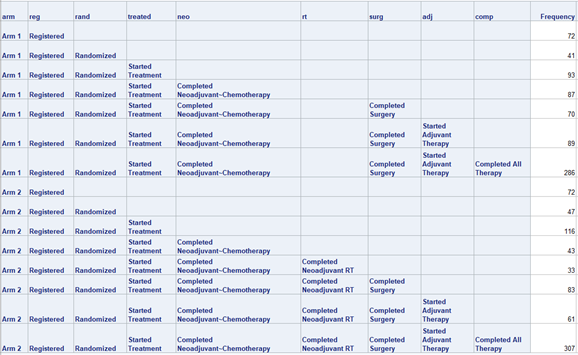Figure 2. The frequency table makes it clear that there are patients that end the study treatment at each node of the CONSORT.

## example macro runs

The %CONSORT macro has the following required parameters:

• DATA: designates the input data set containing the variables to plot
• ID: variable containing the unique identifier for each patient
• NODE: Space delimited list of variables to designate the nodes of the CONSORT

The following is a basic macro call with only the required parameters:

``%CONSORT(DATA=EXAMPLE, ID=ID, NODE=REG RAND TREATED NEO)``

This example only shows the first four nodes because after that point there is a split with multiple paths that requires an additional parameter in the next example.  This basic macro call produces the following graph:

Figure 3 is the basic graph created by only the required parameters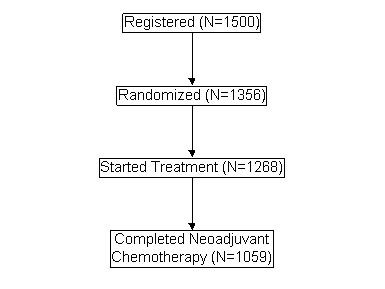Figure 3. The simple CONSORT diagram nodes and counts are automatically created and spaced by the macro program.

There are two other options parameters that are key:

• OFFREASON: Specifies one or more variables that contain the off-treatment reasons
• SPLIT: Specifies a variable to use to split the CONSORT into branching paths.

The following example adds these options to the previous macro call:

``````%CONSORT(DATA=EXAMPLE, ID=ID, NODE=REG RAND TREATED NEO,
SPLIT=|ARM,OFFREASON=OFFTRT|OFFTRT2)``````

The SPLIT variable is assigned to a specific NODE using the | symbol as a delimiter.  In the above example the CONSORT is split by ARM at Randomization instead of Registration due to the first | assigning no SPLIT variable to REG.  The same can be done with OFFREASON and one or more unique variables can be entered for each NODE.  In the case of both SPLIT and OFFREASON the last value is carried forward and does not need to be repeated.  If additional SPLIT variables are listed after the first then the paths will continue to branch.

Figure 4 is the basic graph created by adding the SPLIT and OFFREASON parameters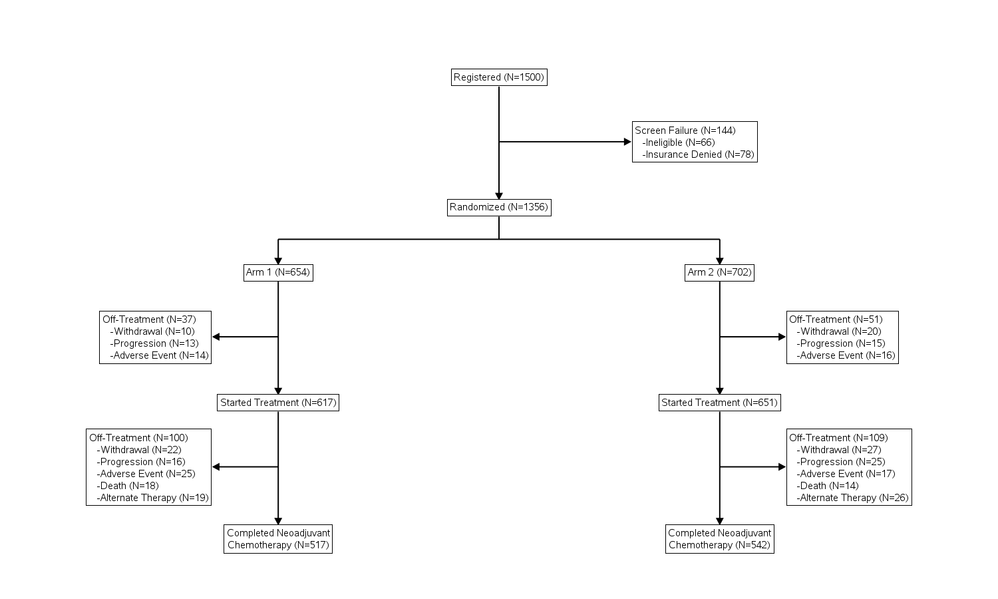Figure 4. The SPLIT variable ARM corresponds to the RAND variable in the NODES list, so the split happens immediately after the RAND row in the diagram.  The OFFREASON boxes are added after each step.  One variable is used between REG and RAND and another between RAND and beyond.

The macro can use as many SPLIT variables as the user provides.  The following example adds in a second SPLIT variable:

``````%CONSORT(DATA=EXAMPLE, ID=ID, NODE=REG RAND TREATED NEO,
SPLIT=|ARM|SEX,OFFREASON=OFFTRT|OFFTRT2)``````

Figure 5 is the graph created by adding a second SPLIT variable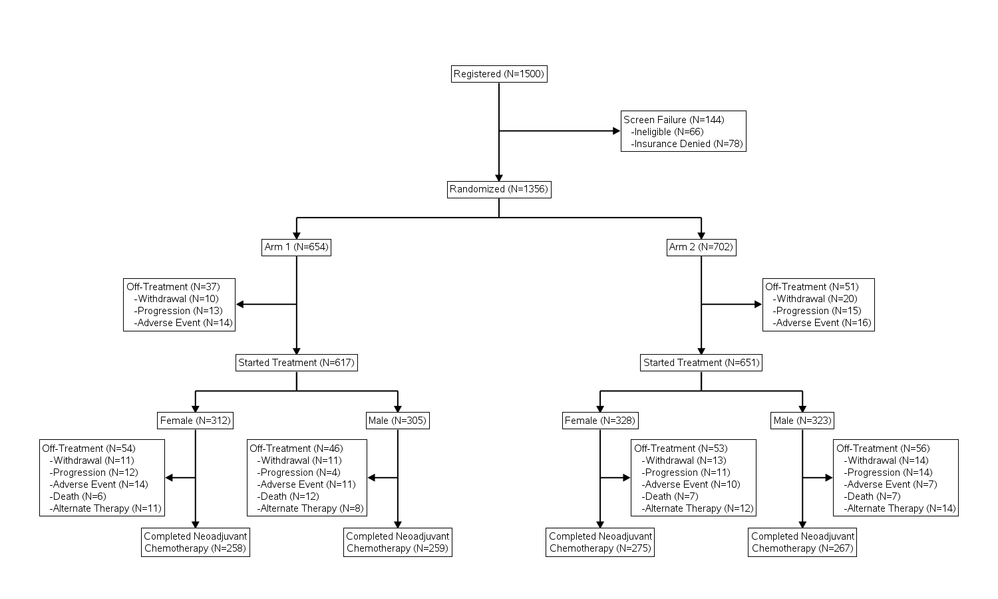Figure 5. The second SPLIT variable, SEX, corresponds to the TREAT variable causing the paths to split the row after TREAT.  There is no limit to the number of SPLIT variables, but space does become an issue.

The CONSORT macro does have other useful parameters for annotation and fine tuning, but the parameters shown in the examples are key to making a basic CONSORT diagram.

## graphical components

The actual creation of the CONSORT diagram is straightforward once the complicated data setup is complete.  There are only two components of the graph:

1. The textboxes containing the values and counts
2. The lines connecting the text boxes to show the flow of the CONSORT

The CONSORT macro uses the SGPLOT procedure to generate the graph.

## Designing the textboxes

The textboxes are created using the TEXT statement which has three required inputs: X (x-coordinate), Y (y-coordinate), and TEXT (text value to be printed at x, y).  There are a few key options used to make the text into a textbox:

• OUTLINE: turns the outline on around the text
• SPLITCHAR: determines one or more text characters to create a new line when encountered.  The CONSORT macro uses the ~ character since it is not commonly used in text strings
• SPLITPOLICY: determines how often the text is split into new lines when encountering the SPLITCHAR characters.  The CONSORT macro sets this to SPLITALWAYS so that it will always create a new line break
• SPLITJUSTIFY: determines if the text will be left, center or right aligned in the textbox.  This is necessary for aligning the off-treatment reasons correctly
• BACKFILL: sets the background of the textboxes to be opaque.  This prevents any connecting lines running behind the text box from being seen.  Setting TRANSPARENCY=0 will make the background fully opaque.
• STRIP: removes trailing and leading blanks from the text values

The POSITION option determines which anchor point around the textbox sits on top of the x/y coordinate.  For example, if POSITION=TOP then the top center point of the textbox matches the x/y coordinates and the text is drawn below it.  The CONSORT macro uses different positions depending on which type of textbox is being drawn.  If the textbox is one of the nodes then POSITION=BOTTOM, and if the textbox is one of the off-treatment reason boxes then POSITION will either be LEFT or RIGHT depending on the direction the textbox pops out from the CONSORT.  There are a couple of odd behaviors that occur with POSITION to be aware of:

• There is an option for POSITION to be set to a character variable instead of a keyword.  There is currently an odd reaction between this and SPLITJUSTIFY such that the SPLITJUSTIFY value is potentially ignored and BOTTOMLEFT is selected instead if SPLITJUSTIFY has a value.
• The POSITION value will always match up to the same point of the textbox regardless of the value of PAD.  This complicates lining up the connecting arrow to the top of the box as the padding must be accounted for so that the arrow is not blocked by the textbox.  Adding an option to anchor on the edge of the box instead would be a great addition by SAS.
Figure 6 is an example showing how the textboxes are anchored around padding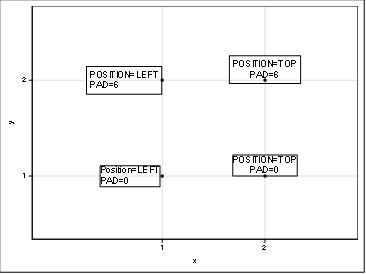Figure 6. The scatter plot points show the actual anchor point and the text stay in the same location regardless of the value of PAD.

The following is an example of the TEXT plot statement within the CONSORT macro:

``````text x=x_b y=y text=start / outline pad=2px position=bottom transparency=0
splitchar='~' splitpolicy=splitalways backfill nomissinggroup
group=label fillattrs=(color=&textbox_background_color)
textattrs=(color=&font_color size=&font_size. pt family="&font") strip
outlineattrs=(thickness=2pt color=&textbox_outline_color)
SPLITJUSTIFY=center;
``````

## Designing the connecting lines

The connecting lines are created with the SERIES statement which requires an x-coordinate and a y-coordinate.  The CONSORT macro uses two different SERIES statements where one has arrowhead caps and the other does not.  The macro ensures two lines do not overlap.  Each line segment in the CONSORT has a starting point, ending point, and unique identifier code.  This code is used in the GROUP option for the SERIES statement to separate them.

When connecting textboxes that are stacked in the same column the coordinates are simply the current textbox anchor point and the previous textbox anchor point.  When the connecting boxes are in different columns, such as when a SPLIT variable is listed, then the macro creates a few separate lines:

• A line starting at the previous textbox anchor point that goes straight down to halfway between the textboxes with no arrowhead
• A line starting at the midpoint between textboxes straight above the current textbox that continues down to the current textbox with an arrowhead
• A line at the midpoint between the textboxes that runs horizontally from the x-coordinate of the previous textbox to the x-coordinate of the current textbox with no arrowhead

These three lines combine to create the branching path shown in the CONSORT diagram.

## Setting up the graph space

The graph space is designed in a simple way such that the rows and columns of the CONSORT can be calculated as a percentage of 100.  The x and y axes both range from 0 to 100 and both axes have all display turned off in the final image.

The vertical space allocated to each row of textboxes is 100/Number of total rows by default.  There is another option in the macro to make the space allocated to each row proportionate to the maximum number of rows of text within that row compared to the rows of text across all rows.  This can help the off-treatment reason boxes fit better when many rows are present.

The horizontal space is allocated equally across all columns.  A column is defined as having the same x-coordinate across the textboxes.  The off-treatment text boxes are not included as columns in this definition and are instead placed at the midpoint between text boxes with optional adjustment available in the macro options.

## Manually designing the consort

Manually setting up the simple components of the CONSORT is straightforward but requires many attempts to get the spacing correct.  The challenge is creating a way to automate the process to remove the tedium of trial and error as much as possible.

# Macro automation methods

There are 4 major items that must be determined by the macro in order to automate the CONSORT:

1. The number of unique paths the patients follow through the trial
2. The parent-child relationship between textboxes in each path
3. The counts and values within each textbox
4. The x/y coordinates of the text boxes for plotting and creating connecting lines

Once these four items are computed by the macro the data set needed to plot the CONSORT can be created.  The CONSORT macro relies heavily on the SQL procedure for merging data and aggregating counts.  The DATA step is also used primarily for array functionality.

## Finding the number of unique paths

The primary purpose of requiring the input data set to have NODEs as multiple variables is that it is more straightforward to find the correct sequence order.  Having nodes represented by multiple rows would require a separate variable or option to determine the correct order.  The first thing the CONSORT macro does is to “transpose” a copy of the input data set into a format that is conducive both for summarizing with the SQL procedure and for painting a picture of each patient’s individual path through the study.

### “Transposing” the data set

The data input into the macro is initially one row-per-patient with multiple variables for each node of the CONSORT.  The macro will cycle through each of the NODE variables, utilize the patients that have non-missing values, and output that as a new step for that patient.  There are two occurrences that would also add another step for each patient:

1. There is a SPLIT variable added at the current node.  This tells the macro to output the node as normal as a “header” for the split, and then to output another row of data for the patient including the SPLIT variable’s value.
2. The patient does not have the current node but has the previous node. An additional row is added with the current off-treatment reason and label.
1. NOTE: it is possible for paths to have nodes that other paths do not such as the EXAMPLE data set does.  If this is the case and a patient continues after skipping a node then only the last off-treatment reason is kept if the patient does not complete the study.

Figure 7 shows patient 19’s data in the input data set and the “transposed” version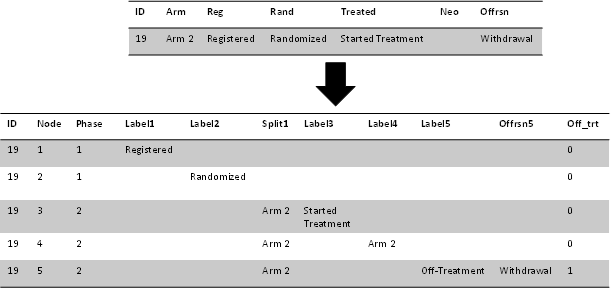Figure 7. The NODE variable represents the current node of the CONSORT and is used for sorting.  Each node row has its own potential set of variables such as LABEL and OFFRSN.  These variables are later combined into one variable.

Figure 7 has a simplified version of the transposed data set focusing on one patient.  With more patients there are potentially more nodes due to patients going off-treatment at different points.  The data is initially setup this way for two reasons:

1. The different NODE, SPLIT, and OFFRSN variables potentially being different data types.  They could be combined with more front-end effort, but it is easier to combine later with COALESCEC and VVALUE functions
2. The variables potentially have different formats which are preserved for ordering

Another data step collapses the multiple variables into one. The NODE variable essentially represents the current row of the CONSORT counting top to bottom.  The PHASE variable increasing in value indicates that a new SPLIT variable has been added to the CONSORT, and the OFF_TRT variable is a flag indicator variable to mark the row as an off-treatment section.

The next steps create an ORDER variable based off the sorted values of all the SPLIT variables entered into the macro across the nodes.  This ORDER variable is combined with the NODE variable to create a unique NODE value for each potential textbox.

Figure 8 shows patient 19’s data after ordering and collapsing the variables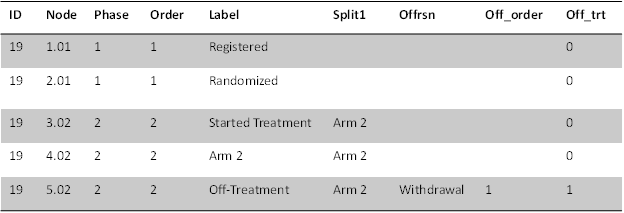Figure 8. The ORDER variable is the order of all SPLIT variable levels including missing values.  This value divided by 100 is added to the NODE variable value to create a distinct value for each textbox.

Now that each textbox now has a unique NODE value and unique LABEL (text) value the next step is to find all of the unique patient paths through the study.  This will be done for two different types of paths:

1. Patients that either completed treatment or have not yet come off active treatment
2. Patients that have gone off-treatment prior to completing the protocol

Figure 9 shows the unique paths through the CONSORT in Figure 4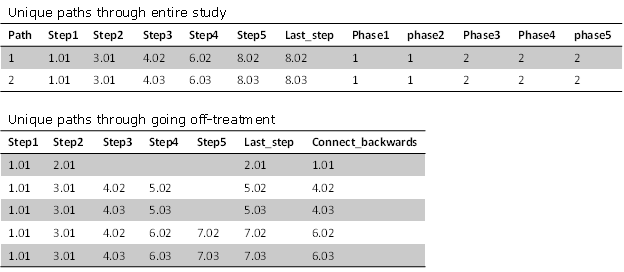Figure 9. The two tables show the unique paths through the study and up-to going off-treatment early.  Each textbox is represented by its unique NODE number

All the unique paths are now contained within these data sets.  The next step is to determine the parent-child relationships between each node.

## Finding the parent-child relationships between nodes

Knowing which nodes connect to each other is key to lining them up into the correct columns and determining which coordinates link when making the connecting lines.  The first step the macro takes is to find the connections (forward and backward) each node makes in order to link the y-coordinates between nodes.  The columns and x-coordinates have not been calculated yet to link.  The following code uses the first data set from Figure 9:

``````data _temp6;
set _unique_paths end=last;
array step {%sysevalf(&ngrps+1)};
array phases {%sysevalf(&ngrps+1)};
retain nsteps;
nsteps=max(nsteps,dim(step)-nmiss(of step(*)));
do i = 1 to dim(step);
if ^missing(step(i)) then do;
phase=phases(i);
node=step(i);
row=int(step(i));
if i=1 then do;
connect_forward=step(i+1);
connect_backward=step(i);
output;
end;
else do;
connect_forward=step(i+1);
connect_backward=step(i-1);
output;
end;
end;
end;
if last then call symputx('nsteps',nsteps);
keep phase path node row row_link connect_forward connect_backward;
run;``````

The &NGRPS macro variable is the maximum number of steps found in any of the unique paths.  The array functionality makes it simple to find the nodes that connect forward or backward and to create a row_link variable that will be used in another data step’s array to find the x/y coordinates of the connecting nodes.   Running the code will create the following data set:

Figure 10 shows the first step of linking rows and nodes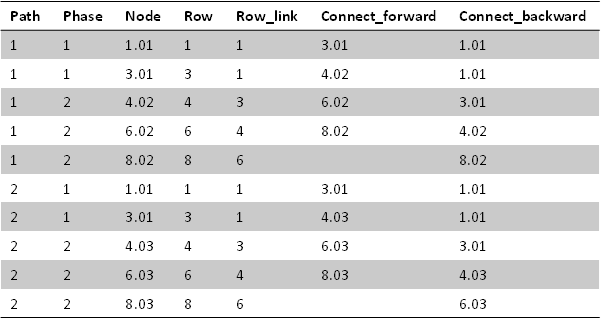Figure 10. The CONNECT_FORWARD and CONNECT_BACKWARD columns are used for merging information about other NODES.  The ROW_LINK and ROW are used as ARRAY indexes in a later data step.

### Calculating the counts and values within the text boxes

The initial steps of calculating the counts and forming the text for the textboxes is easily performed with the SQL procedure and the “transposed” data set from earlier.  The macro makes use of the flag variables created earlier and calculates the counts separately between the nodes and the off-treatment textboxes.  The code for the nodes is:

``````select phase,node,label,'BOTTOM' as position,count(distinct id) as n,
case(missing(label))
when 0 then strip(label)||' (N='||
strip(put(calculated n,12.0))||')'
else '' end as text length=1000
from _temp4 where off_trt<1
group by phase,node,label,position
``````

The code counts the number of unique IDs at each node of the CONSORT and makes a new variable (TEXT) that concatenates the textbox label already in the data set with the new count in a common (N=xx) format.  The where clause uses the OFF_TRT flag variable to exclude the off-treatment boxes from this query.  The off-treatment textboxes are more complex in that they need to have a label with the total count as well as a row for each off-treatment reason with an individual count.  This is done in three steps with the above query using an OUTER UNION CORR to join the four queries together into one final data set _TEMP5 in the macro.  The first step utilizes the overall label and count for the textbox which is stored in the LABEL variable.  The next query takes each off-treatment value which is stored in the OFFRSN variable and then aggregates the counts.  The final query summarizes the counts for any patients that did not continue to the next node but did not have an off-treatment reason.  These patients could be missing the reason or they could still be on active treatment.  The macro labels these patients through the &NO_OFFREASON_TEXT macro variable from the macro call.  The default value for this macro variable is “Active Treatment.”  The off-treatment reasons are indented using another macro option &INDENT_TEXT.  The default is set to be three non-breaking spaces and a dash.  A simplified version of the macro code is:

``````/**Grabs label and total count**/
select phase,node,off_trt,n_off,label, 'RIGHT' as position,
count(distinct id) as n,
strip(label)||' (N='||strip(put(calculated n,12.0))||')' as text
from _temp4 where off_trt>=1 and ^missing(offrsn) and
node ^in(select node from _unique_paths_off
where connect_backward in(select last_step from _unique_paths))
group by phase,node,off_trt,n_off,label,position
outer union corr
/**Grabs each individual non-missing off-treatment reason**/
select phase,node,off_trt,n_off,off_order,offrsn,'RIGHT' as position,
count(distinct id) as n,
&indent_text||strip(offrsn)||' (N='||
strip(put(calculated n,12.0))||')' as text
from _temp4 where off_trt>=1 and ^missing(offrsn) and
node ^in(select node from _unique_paths_off
where connect_backward in(select last_step from _unique_paths))
group by phase,node,off_trt,n_off,off_order,offrsn,position
outer union corr
/**Grabs patients that didn’t continue but don’t have a reason**/
select phase,node, 1000 as off_order,offrsn,'RIGHT' as position,
count(distinct id) as n,
"&no_offreason_text (N="||strip(put(calculated n,12.0))||')' as text
from _temp4 where off_trt>=1 and missing(offrsn) and
node ^in(select node from _unique_paths_off
where connect_backward in(select last_step from _unique_paths))
group by phase,node,off_order,offrsn,position
``````

The OFF_ORDER variable keeps the off-treatment reasons in order.  The final dataset is then sorted by PHASE, NODE, and OFF_ORDER.  The created data set paints a visual picture of what will be in the consort diagram.  There are no x/y coordinates yet in the data set, and there are multiple rows for off-treatment nodes that will be collapsed in the final steps of the macro.  The following figure displays the data set made by these steps:

Figure 11 shows the final data set made in this section.Figure 11. The TEXT column contains the combined labels and counts for each node.  The POSITION value will determine which X variable is assigned to the node in the final steps.

### Calculating the x/y coordinates of the text boxes

The next step focuses on finding the x- coordinates for each node.  The program initially gives each node an equal amount of space depending on how many paths exist in that row.  The next steps then use the parent-child links to center each node between its forward connecting nodes.  The simplified process is shown in the following figure:

Figure 12 shows how the x-coordinates start out as evenly spaced and then are adjusted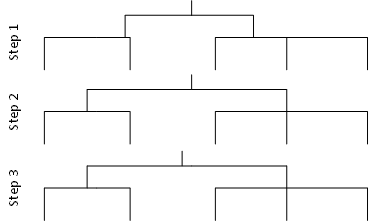Figure 12. In step 1 each branch is simply centered based on how many paths there are in that row.  Then working bottom to top in the next steps center the branch between the forward connecting paths.

The code that assigns the initial x-coordinates is the following:

``````create table _temp7 as
select distinct a.phase,a.node,a.connect_backward,a.connect_forward,
a.min_path,a.max_path,c.npaths,a.total_npath,a.x_min,a.x_max,a.x,a.y
from (select *, count(distinct path) as npath,
min(path) as min_path, max(path) as max_path,
100*(calculated min_path-1)/max(total_npath) as x_min,
calculated x_min+100*count(distinct path)/max(total_npath) as x_max,
(calculated x_max+calculated x_min)/2 as x,int(node) as y
from (select a.*, b.path, b.total_npath _temp6 (drop=path) a
left join  (select *,count(distinct path) as total_npath from _temp6) b
on a.phase=b.phase and a.node=b.node)
group by node) a left join
(select phase,count(distinct node) as npaths from
(select phase,node,path from _temp6
group by path,phase having node=min(node))
group by phase) c
on a.phase=c.phase;
``````

And the resulting data set is:

Figure 13 shows the initial x-coordinates for each node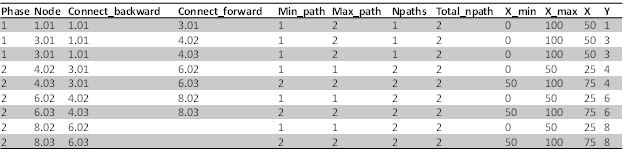Figure 13. The SQL procedure works well for performing multiple merges and on-the-fly data set modifications for this step.

The code that then goes from bottom to top of the CONSORT to reassign the x-coordinates is:

``````    select distinct row into :dsteps separated by '|' from _temp6;
%do i=&nsteps %to 1 %by -1;
create table _step&i as
%if &i=&nsteps %then %do;
select * from _temp7 (drop=connect_forward x_min x_max)
where y=%scan(&dsteps,&i,|);
%end;
%else %do;
select a.phase, a.node, a.connect_backward, a.y,
coalesce(min(b.x),max(a.x_min)) as x_min,
coalesce(max(b.x),max(a.x_max)) as x_max,
coalesce((min(b.x)+max(b.x))/2,max(a.x)) as x
from (select * from _temp7
where y=%scan(&dsteps,&i,|)) a
left join _step%eval(&i+1) b on a.connect_forward=b.node
group by a.phase, a.node,a.connect_backward,a.y;
%end;
%end;``````

The current example would not change since it has a balanced number of branches on each side of the CONSORT, but the output data set would be nearly the same as Figure 13 with updated x-coordinates.  Each unique x-coordinate is counted as a new column in ascending order.

The y-coordinates are much easier to calculate, and there are two methods.  The first counts the total number of rows in the CONSORT data (Figure 10) and then assigns an equal amount of vertical spacing for each row (1/&NROWS).  The y-coordinate would then be the center of each set of vertical space.  The second is a more flexible method that counts maximum number of text lines in each row of nodes.  The denominator then becomes the total number of lines across all rows (maximum of each row) to assign the vertical space.

## FINALIZING THE PLOT DATASET

Now that all the data pieces were calculated by the macro, they are merged into a final preparation data set.  There are still multiple rows for off-treatment nodes that must be collapsed, and this is done in the following data step:

``````data _temp9;
set _temp8;
by y x;
where ^missing(x) and ^missing(y);
length _temp_text \$10000.;
if first.x then call missing(_temp_text);
if ^(first.x and last.x) then do;
_temp_text=catx('~',_temp_text,text);
if last.x then do;
text=_temp_text;
output;
end;
end;
else output;
retain _temp_text;
drop _temp_text;
run;``````

The program creates a text variable that concatenates the off-treatment label with each row of the off-treatment reasons.  The final output only has the final row of each node with the concatenated text variable:

Figure 14 shows the data set with the concatenated text variableFigure 14. The final data set has the text value, the y value that will be converted to a y-coordinate, the x-coordinate, and row/column indexes for arrays in the next steps.

The next step sets up a data set with attributes of each textbox (x/y-coordinates, number of text lines, and position) all in the same row so that they can be used in arrays.  This data step is then merged into the data set from Figure 14 so that each row has access to the attributes of all the other textboxes.

The last components needed for the plot are the coordinates for the lines.  There are several that must be considered.  The components that are needed to make each line:

• Two x/y coordinates: each line must have a start and an end
• Unique ID value to be used in the GROUP option to keep all lines individual

## The linking textboxes are in the same column

This is the simplest scenario with the most straightforward calculation.  This scenario only requires a straight line from the x/y coordinates of the previous textbox to the x/y coordinates of the current textbox.  This will cause the line to run behind the previous textbox as the anchor points are at the top and middle of the textbox, but because each textbox is opaque that part of the line will not be seen.

## The linking textboxes are in different columns and position=”Bottom”

This is the scenario when a SPLIT has occurred in the prior row of the CONSORT.  The macro moves the connecting lines in a rectangular path rather than at an angle (see Figure 4).  Due to this there are three lines that need to be created:

1. A line that runs from the vertical midpoint between the two textboxes to the current textbox with an arrowhead.  The x-coordinate for this line is the same as the current textbox for both points
2. A line that runs from the previous textbox to the vertical midpoint with no arrowhead.  The x-coordinate for this line is the same as the previous textbox for both points.
3. A line that runs horizontally at the vertical midpoint from the x-coordinate of the current textbox to the prior textbox with no arrowhead

While the above seems straightforward there is one major complication: the vertical midpoint between textboxes is from the bottom of the previous textbox to the top of the current textbox.  The x/y coordinates are known for the top of the current textbox but the y-coordinate of the previous textbox is not directly available and must be estimated.  The CONSORT macro has the macro parameter &MULTILINE_ADJUST to assign a certain amount of space for each line of text, and this is used to estimate the distance from the top of a textbox to the bottom:

The macro parameter can be adjusted depending on font size to approximate the bottom of the textbox, and this estimate can then be used to calculate the vertical midpoint.

## The linking textboxes are in different columns and position^=”Bottom”

This scenario applies to the off-treatment textboxes which have a position of LEFT or RIGHT.  They are designed to pop out at the vertical midpoint between the previous textbox and the following textbox.  This y-coordinate is computed the same way as the previous example, but uses the previous and following textboxes instead of the current one.  The x-coordinates for this line are the x-coordinate of the previous textbox and the x-coordinate of the current textbox.

Figure 15 shows an example of the calculated x/y coordinates for linesFigure 15. There are two separate sets of x/y variables for lines.  The x_line/y_line combinations are for lines with arrowheads, and the x_line2/y_line2 combinations are for lines without arrowheads.

The SGPLOT procedure runs multiple TEXT statements and multiple SERIES statements which requires different x variables to use in the different statements.  The X_B variable is for TEXT statements with POSITION=BOTTOM, X_L for POSITION=LEFT, and X_R for POSITION=RIGHT.  The ID variable is used with the SERIES plot statements, and there are two sets of x/y coordinates for each ID value.  In Figure 15 above, the ID combinations for 1004 show the three sets of lines that occur when a SPLIT variable is used.

## Conclusion

The concept of automating a CONSORT diagram programmatically was extremely challenging.  There are many, many ways to design a CONSORT diagram and it is nearly impossible to write one program that can cover them all.  The methods described in this paper, however, can be applied to any CONSORT program with modifications for the final product.  There are numerous other functions and features of the CONSORT macro that were not described by this paper because focusing on the more universal methods behind the macro.

## References

1Matange, Sanjay and Hebbar, Preshant. “CONSORT Diagrams with SG Procedures.” Proceedings of the PharmaSUG 2018 Conference, Seattle, Washington

## Acknowledgments

A special thanks to the current and former SAS ODS Graphics team including Preshant, Sanjay, and Dan for always being willing to discuss ideas and giving encouragement.  Another thank you to SAS technical support for helping me find solutions for potential SAS glitches while making this program.

## Contact Information

Your comments and questions are valued and encouraged. Contact the author at:

Jeffrey Meyers

Mayo Clinic

Meyers.jeffrey@mayo.edu or jpmeyers.spa@gmail.com

SAS and all other SAS Institute Inc. product or service names are registered trademarks or trademarks of SAS Institute Inc. in the USA and other countries. (r) indicates USA registration.

Other brand and product names are trademarks of their respective companies.Dear @JeffMeyers ,
I have some questions that I hope you might be able to assist me in answering.
When I used the code you attached, the result was not show same with your paper.
Mine figure showed the lable of total but the paper showed not have the lable of total.
Beside that, the offtrt2 label also can't show properly.
I had try by using SAS 9.4 and also Studio.
Could you provide me with some guidance about how to solve this issue?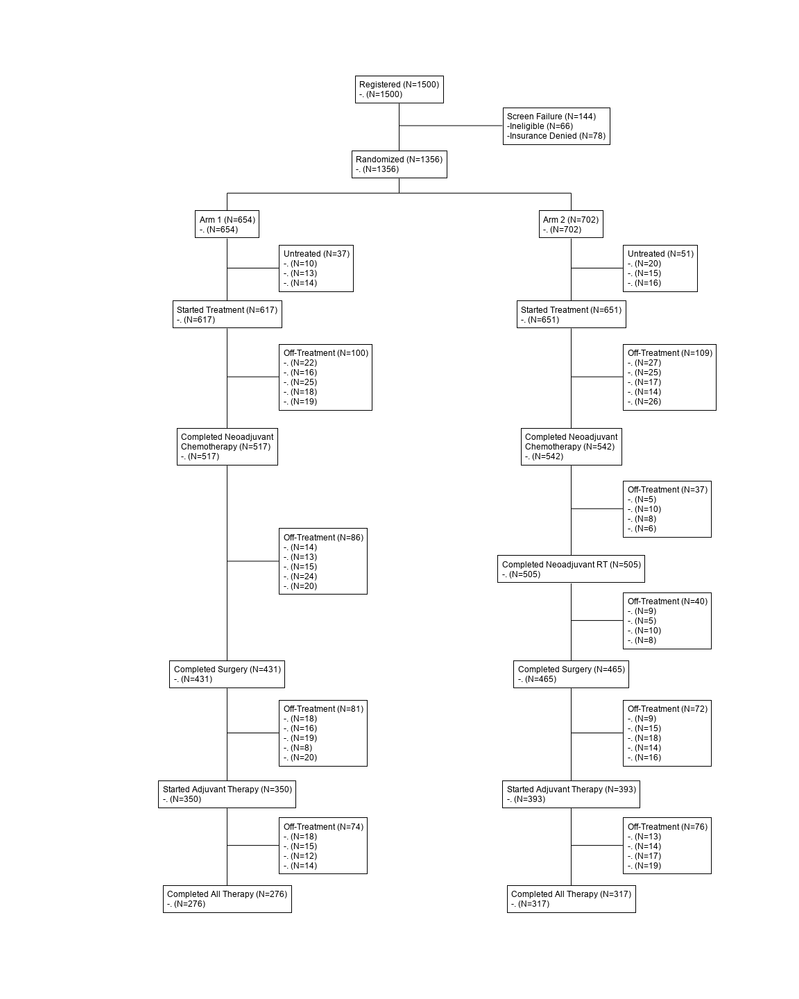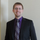Hello @erynwu ,

I recently changed positions and at my new employer had a completely new system/SAS version.  As I've been testing this macro I've come to realize how much difference the platform and SAS version can make.  Can you let me know what platform (Windows/Linux/Etc.), version and application you're using to test run?  It looks very strange as the reasons are seen as different so much as to get counts, but still all showing up as .'s every where except for the screen failures.  I have a feeling you might be running this in a Windows platform as the way it detects/shows missing is different than Linux (where I wrote this).  I'm going to suggest e-mailing me at jpmeyers.spa@gmail.com to get more details/discussion.Hello @erynwu & @JeffMeyers,

I ran into the same issue as erynwu when running the macro on on SAS Enterprise Guide v8.2 Update 2 (8.2.2.1255) on Windows 10.  I believe I found the root cause.

The columns used to specify the offreason and or freq marco parameters are numeric. As a result the default missing values are represented by a period. When these values are processed into character strings, the period is carrying over instead of creating a blank character cell. The subsequent steps in the macro treat cells with a "." the same as a if they held a legitimate value (e.g. "Insurance Denied").

I was able to fix the issue by specifying the default missing value to be blank:

`options missing="";`

If that doesn't work for your particular SAS deployment/environment, you'll likely have to update the macro itself to remove periods from the offrsn and freq columns in the temporary tables. The compress function should work for this. The examples below would keep only the alphabetic characters of a string:

```compress(offrsn,,'ka')
compress(freq,,'ka') ```

Thanks for developing this macro @JeffMeyers!  It's been very helpful.

Version history
Last update:
‎11-23-2021 11:53 AM
Updated by:Contributors
Article Labels
Article Tags